# SSL Minutes

15 Feb 2001
Boytchoavle will be the Beverage Person for Tuesday next.achel said something about brownies.

## Intro to Ergodic theory

This is a theory which studies measurable dynamical systems. Being measurable means that said systems are probabilistic.

## Simple example: Fair Coin

If we throw a coin repeatedly we can talk about the probability of a sequence of events. For instance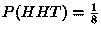Some Markov Chains also exhibit ergodicity. A simple MC is for instance: If it rains today then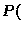it will rain tomorrow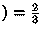, and if it is sunny today thenit will be sunny tomorrow.

The above examples have one dimensional time, but that need not necessarily be the case with ergodic systems. We can have two (, three, &c.) dimensions of time. For example see the domino tiling in fig 1.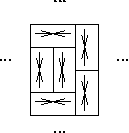Here we use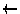to denote one of four possible states. The system depicted above has some built in restrictions. A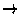state can only be to the left of asquare. A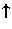state can only be under a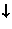square.

An Interesting Question is: How random can a system be with restrictions such as the above?''

Entropy is a measure of randomness.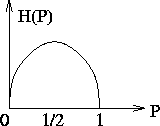The figure above illustrates entropy (denoted traditionally by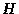for some reason), in the case of a coin. If the coin is biased so that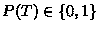, then there be no randomness, thus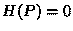. If the coin is fair, i.e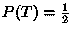then we have maximum entropy.

If we are taking measurements of a sequence of events (say repeated tossings of a coin) at the rate of one measurement per unit of time, the most randomness we can hope for is 1 bit per unit of time.

Then there was a brief discussion of Independent Identically Distributed events. The author of these notes is not quite certain, how to tie that in with the rest of the discussion, yet it seemed relevant enough to be mentioned.

## Another example

of a one dimensional system is the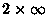square grid tiled by dominoes. A piece of it is shown in the figure bellow.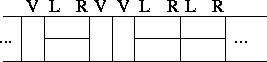In this case a restriction is that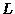and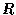states must be next to each other in the correct order. (There are exactly two tilings that we are neglecting here for their negligably low probability of occurring. Those are the two brick-work'' tilings). Here we prefer to use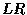for horizontal dominoes as opposed to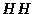, for this makes it a local rule, and makes it easier to verify if a piece of the tiling is correct. It takes a while to check if a long sequence ofs is of even length.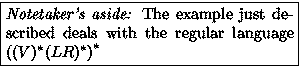So a question we want to answer is how random can a piece of that strip be?

If we start somewhere on the strip we want to know how likely are we to see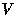in the next column as opposed to?

One way to make a random system of this kind is to use a biased coin to decide whether to put down aor an. If the bias-ratio is too close to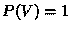(always) or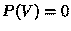(always LR), the system will have low entropy. It has higher entropy when the bias is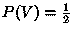(a fair coin), but this is still not the best choice. It turns out that the choice of bias that leads to the highest possible entropy is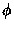(aka The Golden Ratio).

There is a close relationship between the Golden Ratio and Fibonacci numbers, namely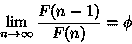in English: The ratio of two successive Fibonacci numbers approaches.

Now we can think back to the first homework for some insight on why this is a good bias for this particular coin. Consider a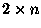piece of the strip. The first column of it can beor. If it bewe have a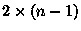piece to tile, otherwise the untiled part is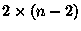. As we have seen the probabilities involved are related to the Fibonacci numbers.

Hal has seen matrices related to a similar system.

## In the 2-dimensional case

we can think of covering the plane with dominoes. One simple way to go about that is to divide the plane in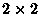pieces and use a fair coin to tile each of these pieces. We then have 1 bit of information to each, therefore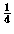bit per square. And we can do much better than that.

If we take a random tiling of a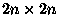square with dominoes, the probabilities of what domino covers a square in the inside are very much the same as they are in the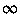-plane. We can ask for instance how likely are we to come across three horizontal dominoes, stacked one on top of another in a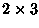. The situations would again be similar on a largeand the entire plane.

If we take however and Aztec Diamond, things go terribly wrong really fast. In the polar regions for instance the probabilities are skewed at places that are much further away from the border than in the simple square case.

In the very center of the Aztec Diamond, we have the max entropy.

We can use the Generalized-Domino-Shuffling (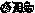) algorithm to find the probability of a stack of three h-dominoes by finding the number of matchings of a graph depicted below.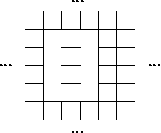If we chose to leave all the edges between the six vertices of interest as to simplify the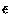calculations in, we would increase the number of matchings by a factor of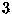.

In the Aztec Diamond, the polar regions are 0-entropy regions, whereas the temperate zone is of positive entropy.

If we travel from the center of the Aztec Diamond towards one of the poles, at about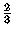of the way the probability of having a north'' domino approaches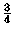.

Another phenomenon that may occur is Hole in The Wall''. This is a rectangle of even width, which if it is shaded in standard checkerboard fashion, has the white squares from the leftmost column and the shaded squares from the rightmost column removed. In other words, the sizes of consecutive rows alternate between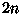and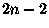and the rows are centered left to right. Such an animal has an unique tiling by dominoes.

The point of this is, that we may achieve the behavior of the sub-arctic region of an Aztec Diamond on a square if we remove the right pieces from the border.

Then we talked about halved Aztec Diamonds, and lattice paths in them, which force tilings.

Then Prof. Propp addressed a question by yours truly having to do with the basic moves on a Diabolo-tilable region. An appropriate illustration of the graph that we would want to match can be seen on p.10 of thepaper. The graph which consists of octagons and squares. The point is there are TWO basic move in the Diabolo world, one deals with the two matchings of a square, the other with the two matchings on an octagon.

The question was raised as to how to get a text file to fit predetermined line lengths in UNIX, which brings us to The UNIX Command of the Day: fold(1).